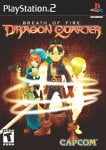# D-Ratio FAQ - Guide for Breath of Fire: Dragon Quarter

Scroll down to read our guide named "D-Ratio FAQ" for Breath of Fire: Dragon Quarter on PlayStation 2 (PS2), or click the above links for more cheats.```-- Breath of Fire V D-Ratio FAQ --
-- By Kouli {aka Ken, [email protected]} --
-- Final Version --

----------------------------------------------------------------------------------
--

What is D-Ratio? First of all, this is not D-Counter. D-Ratio is sort of like a
rank
that you get upon beating the game. When you beat the game, you will recieve a
D-Ratio that is based on the catagories below. With higher D-Ratio, you can
unlock
doors that you couldn't open when you have low D-Ratio. Ryu starts the game with
D-Ratio 1/8192.

----------------------------------------------------------------------------------
--

The D-Ratio calculation splits into A, B, C, D, E, F and G catagories:

A} Game Clear Time

Within 8hrs  = 10 Pts
8 -  9hrs  =  9 pts
9 - 10hrs  =  8 pts
10 - 11hrs  =  7 pts
11 - 13hrs  =  6 pts
13 - 15hrs  =  5 pts
15 - 17hrs  =  4 pts
17 - 20hrs  =  3 pts
20 - 23hrs  =  2 pts
23 - 26hrs  =  1 pt
26 - higher =  0 pt

B} EX TURN Acquisition Percentage

* To obtain an Extra Turn, simply hit the enemy before it touches you

95 - 100% = 10 pts
90 -  95% =  9 pts
85 -  90% =  8 pts
80 -  85% =  7 pts
75 -  80% =  6 pts
70 -  75% =  5 pts
65 -  70% =  4 pts
60 -  65% =  3 pts
55 -  60% =  2 pts
50 -  55% =  1 pt
Below 50% =  0 pt

C} Map Completion Percentage

98 - 100% = 10 pts
96 -  98% =  9 pts
94 -  96% =  8 pts
92 -  94% =  7 pts
90 -  92% =  6 pts
88 -  90% =  5 pts
86 -  88% =  4 pts
84 -  86% =  3 pts
82 -  84% =  2 pts
80 -  82% =  1 pt
Below 80% =  0 pt

D} Number of Opened Treasure Box

* The ones in Secret Dungeon Kokon Horay do not count.

Above 42 = 10 pts
39 - 41  =  9 pts
36 - 38  =  8 pts
33 - 35  =  7 pts
30 - 32  =  6 pts
25 - 29  =  5 pts
20 - 24  =  4 pts
15 - 19  =  3 pts
10 - 14  =  2 pts
5 - 9   =  1 pt
Below 4  =  0 pt

E} Number of Saves

0     = 10 pts
1 - 2   =  9 pts
2 - 3   =  8 pts
3 - 4   =  7 pts
4 - 6   =  6 pts
6 - 8   =  5 pts
8 - 10  =  4 pts
10 - 12  =  3 pts
12 - 14  =  2 pts
14 - 16  =  1 pt
Above 16 =  0 pt

F} Secret Dungeon Kokon Horay

* To get there, you have to get the ants to dig to the lowest level of Fairy
Colony. It's way below the Entrance Room.

B50F     = 10 pts
B46F - B49F =  9 pts
B41F - B45F =  8 pts
B36F - B40F =  7 pts
B31F - B35F =  6 pts
B26F - B30F =  5 pts
B21F - B25F =  4 pts
B16F - B20F =  3 pts
B11F - B15F =  2 pts
B6F  - B10F =  1 pt
Below B5F   =  0 pt

G} Game Clear Level

This catagory is different, all you need to do is to add Ryu, Nina and Lin's
levels
and divided by 3.

Example: Ryu is Level 60 when you beat the game, Nina is Level 60 and Lin is
Level
60, {60+60+60}/3= 60

But the maximum point you can get for this catagory is 50 points, so It's will be
50 points in this case.

----------------------------------------------------------------------------------
--

Finally, You add the point you get from A, B, C, D, E, F and G catagories.

D-Ratio:

100 pts     = 1/4
95 pts - 99.99 = 1/8
90 pts - 94.99 = 1/16
85 pts - 89.99 = 1/32
80 pts - 84.99 = 1/64
75 pts - 79.99 = 1/128
70 pts - 74.99 = 1/256
65 pts - 69.99 = 1/512
60 pts - 64.99 = 1/1024
55 pts - 59.99 = 1/2048
50 pts - 54.99 = 1/4096
Below 50 pts  = 1/8192

----------------------------------------------------------------------------------
--

----------------------------------------------------------------------------------
--

-Me
-CJayC
-Japanese BBS
-Jerel{aka, Genji Masamune}

----------------------------------------------------------------------------------
--

This Document Copyright 2003 Kouli
```